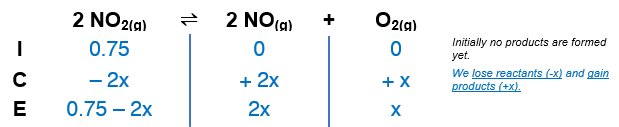# Problem: Nitrogen dioxide decomposes according to the reaction2 NO2(g) ⇌ 2 NO(g) + O2(g)where Kp = 4.48×10−13 at a certain temperature. If 0.75 atm of NO2 is added to a container and allowed to come to equilibrium, what are the equilibrium partial pressures of NO(g) and O2(g)?

###### FREE Expert Solution

We’re given the following equilibrium reaction:

2 NO2(g) ⇌ 2 NO(g) + O2(g)Kp = 4.48×10−13

Construct an ICE table:The Kp expression for the reaction is:

$\overline{){{\mathbf{K}}}_{{\mathbf{p}}}{\mathbf{=}}\frac{\mathbf{products}}{\mathbf{reactants}}{\mathbf{=}}\frac{{{\mathbf{P}}_{\mathbf{NO}}}^{\mathbf{2}}{\mathbf{P}}_{{\mathbf{O}}_{\mathbf{2}}}}{{{\mathbf{P}}_{{\mathbf{NO}}_{\mathbf{2}}}}^{\mathbf{2}}}}$

88% (403 ratings)###### Problem Details

Nitrogen dioxide decomposes according to the reaction
2 NO2(g) ⇌ 2 NO(g) + O2(g)
where Kp = 4.48×10−13 at a certain temperature. If 0.75 atm of NO2 is added to a container and allowed to come to equilibrium, what are the equilibrium partial pressures of NO(g) and O2(g)?